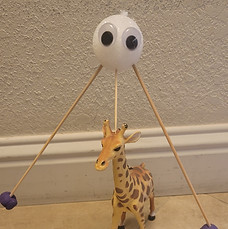top of page# PHYSICSWant to learn about Forces and motions? Light, Sound, Gravity, Magnetism, and Newton's three laws of motion are just a few of the subjects we will cover in these classes.  With hands-on experiments we will discover the fun in Physics and answer questions from our natural world.

4 different sessions to choose from.## Physics Time

Lesson 1      Gravity and Air Resistance

Lesson 2      Light

Lesson 3      Magnetism

Lesson 4      Sound

Lesson 5      Newton's 3 laws of Motion## More Physics

Lesson 1      Balance Forces

Lesson 2      Friction

Lesson 3      Electrical Circuits

Lesson 4      Momentum

Lesson 5      Buoyancy## Balance Twist and Spin

Lesson 1      Whirligig

Lesson 2      Spin Art

Lesson 3      Spin It!

Lesson 4      Balance Bot

Lesson 5      Hurdling and Domino Effect## Forces of Nature

Lesson 1      Air Pressure

Lesson 2      Balance Forces

Lesson 3      Pom Pom Slingshot

Lesson 4      Static Electricity

Lesson 5      Temporary Magnets

bottom of page